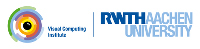OpenMesh
Mesh Decimation Framework

The mesh decimation framework has 3 building blocks.

# The decimation algorithm

The decimater (OpenMesh::Decimater::DecimaterT) provides the decimation algorithm, while the decimation modules provide the computational part. The modules compute a priority value due to some error metric, which is used by the decimater to feed a priority queue. The lower the error value, the more a potential collapse moves to the front of the queue. The one with the lowest error will always be the candidate for the next collapse.

This implementation does a halfedge collapse, hence simply collapsing one vertex into another connected by a halfedge.

Note
The decimater ignores all 'locked' and 'deleted' vertices (see OpenMesh::Attributes::StatusBits)
Attention
The decimater sets temporarily the status bit 'tagged' and clears it after usage regardless of a previous state.

# Block vertices from beeing touched by the Decimater

You could mark vertices as locked, which should not be modified by the decimater.

// That might be already requested
mesh_->request_vertex_status();
// Get an iterator over all halfedges
Mesh::HalfedgeIter he_it, he_end=mesh_->halfedges_end();
// If halfedge is boundary, lock the corresponding vertices
for (he_it = mesh_->halfedges_begin(); he_it != he_end ; ++he_it)
if (mesh_->is_boundary(*he_it) ) {
mesh_->status(_mesh->to_vertex_handle(*he_it)).set_locked(true);
mesh_->status(_mesh->from_vertex_handle(*he_it)).set_locked(true);
}

# Decimating Modules

The vertex to be removed is determined by a decimation module, which has to be derived from OpenMesh::Decimater::ModBaseT. The framework supplies already a few decimation modules. But it's very easy to build your own (OpenMesh::Decimater::ModBaseT). The most important function of a decimation module is OpenMesh::Decimater::ModBaseT::collapse_priority(). It takes an OpenMesh::Decimater::CollapseInfoT describing a potential halfedge collapse, and returns a value due to some error metric. The error value is used by the decimater to feed a priority queue. Collapses with low error will be executed first, and those with large error later. Of course a module computing the error quadric is provided (OpenMesh::Decimater::ModQuadricT).

This framework allows to use more than one decimation module with some restrictions. Since the error value is always normalized and sometimes very difficult to compare to other metrics, the framework allows only one non-binary module, i.e. a module computing a float value. Every further module must be a binary module, i.e. collapse_prioerity() returns OpenMesh::Decimater::ModBaseT::LEGAL_COLLAPSE or OpenMesh::Decimater::ModBaseT::ILLEGAL_COLLAPSE. In the algorithm the binary modules are evaluated first. If the evaluated collapse passes the test, then the non-binary module contributes to the decision step.

In some cases the module does not contribute anything to the decision engine of the decimater, but instead, e.g. simply collects information, while the decimater does it's work. For instance the module OpenMesh::Decimater::ModProgMeshT collects information from all collapses that have been done. This information can be used to generate progressive meshes as described in "Progressive meshes", Hoppe, 1996.

Provided decimation modules(Binary: B, Continuous: C, Special: X):

# Module Handles

Similar to properties the modules are represented outside the decimater by module handles. Before using the decimater a non-binary module must be registrated with the decimater.

See Basic Setup.

# Basic Setup

The following small example show the basic steps to setup up a decimater:

//
using namespace OpenMesh
// ---------------------------------------- necessary types
// Mesh type
// Decimater type
typedef Decimater::DecimaterT< Mesh > Decimater;
// Decimation Module Handle type
// ---------------------------------------- decimater setup
Mesh mesh; // a mesh object
Decimater decimater(mesh); // a decimater object, connected to a mesh